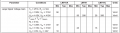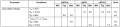# Open loop Op Amp, measuring voltage gain versus frequency

#### rubberducky84

Joined Nov 17, 2017
2
I designed an open loop op amp to measure the gain of a 741 amp. I'm not sure what is wrong here but I don't get an answer that I expect. Is the gain (A) given by A = (1+R2/R1)*Vin ? Measurements are taken from input and output using an oscilloscope, and a signal generator as the input.
What should I expect the gain to be if I send say 20 Hz in the input?

#### MrChips

Joined Oct 2, 2009
29,848
Look at your formula again. Do a dimensional analysis of the formula.
On the left hand side of the equal sign is gain (A) which is a dimensionless quantity.
On the right hand side of the equal sign is volts.
Hence that formula cannot be correct.

#### dl324

Joined Mar 30, 2015
16,146
Welcome to AAC!

What gain did you measure?

What is the amplitude of the input signal?

What open loop gain do you expect?

#### rubberducky84

Joined Nov 17, 2017
2
The formula I gave is for Vout. The gain for an open loop is given by A = Vout/(Va-Vb), where voltages Va= inverting terminal and Vb=non-inverting terminal.
Here's a theoretical case, if I send 2 mV in the input, Va=Vin*(R2 / (R1+R2))=0.002*(33/100033)=6.59e-7V. Then lets say Vb=0 and Vout = 15V, then the gain is
A = 15V/6.59e-7V = 2.27e7.
Does this gain seem right?

The measurements I had before are wrong, so I'm redoing this.

#### BobTPH

Joined Jun 5, 2013
8,104
What is the input bias current of your 741? What is the current into the inverting input? Do you see a problem there?

Bob

#### dl324

Joined Mar 30, 2015
16,146
Here's a theoretical case, if I send 2 mV in the input, Va=Vin*(R2 / (R1+R2))=0.002*(33/100033)=6.59e-7V.
You are giving the equation for calculating the input voltage after the voltage divider.

That has nothing to do with the output. Since you are operating the opamp open loop, gain is determined by the open loop gain which, according to the datasheet, varies from 50-200+(EDIT: V/mV):Opamps aren't very useful when operated open loop.

You'll also have problems with input offset voltage:Last edited:
•absf

#### WBahn

Joined Mar 31, 2012
29,510
Units count -- the open loop gain is spec'ed at between 50,000 and 200,000 (note the units of V/mV, which is equal to 1,000).

•absf